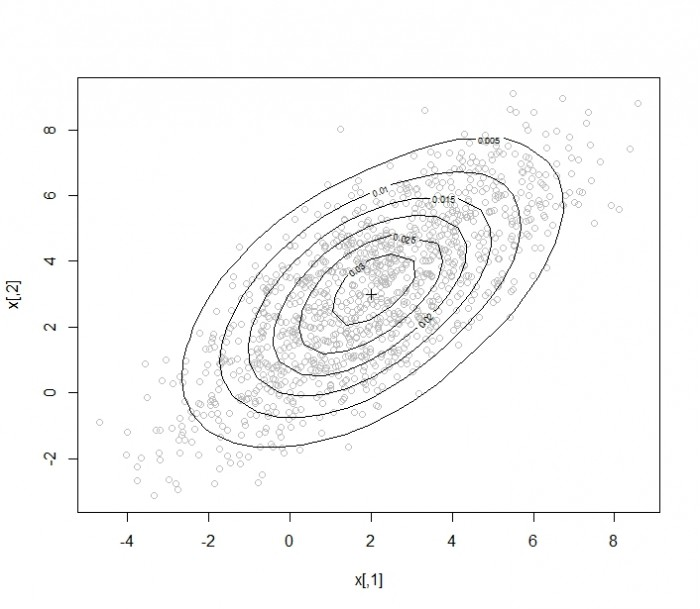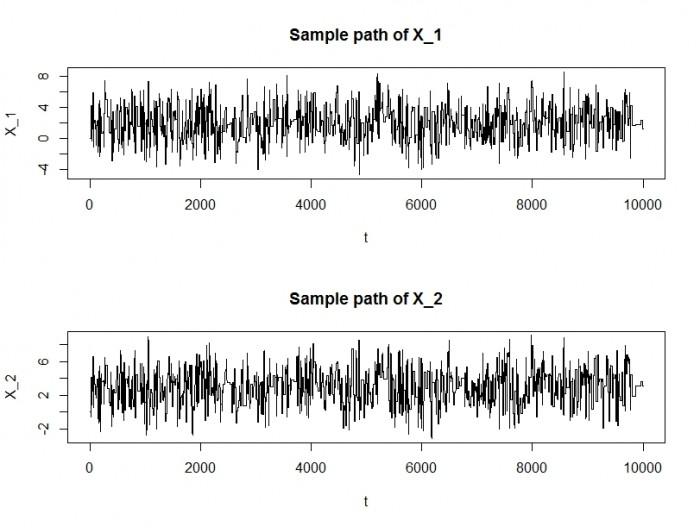MCMC中的Metropolis Hastings抽样法

8,583

Metropolis Hasting（下面简称MH）是蒙特卡罗马尔科夫链中一种重要的抽样方法。本文简要介绍MH算法，并给出一个实例。

MH算法在参数空间随机取值，作为起始点。按照参数的概率分布生成随机的参数，按照这一系列参数的组合，计算当前点的概率密度。依据当前点和起始点概率密度比值是否大于(0,1)之间的随机数来判断是否保留当前点。若当前点的概率密度大于该随机数，就称这种状态为接受状态，此时，在满足参数概率分布的前提下，继续随机抽取参数的组合，作为下一点，计算下一点的概率密度，并计算下一点概率密度和概率密度的比值，并继续循环。若当前点不能被接受，则继续在满足参数概率分布的前提下，继续生成随机数，作为新的参数组合，直到参数组合能够被接受为止。

## 解答：
mvdnorm <- function(x, mu, sigma){
#从x减去mu
x.minus.mu <- x - mu
exp.arg <- -0.5 * sum(x.minus.mu * solve(sigma, x.minus.mu))
# det(sigma) sigma 的行列式
return( 1 / (2 * pi * sqrt(det(sigma))) * exp(exp.arg) )
}

## 问题二
## 假设二元正态分布的参数如下：
## 两个维度的平均值分别为 2， 3
# 协方差矩阵为
# 4 1
# 1 4
# 尝试用蒙特卡洛马尔科夫链 Metropolis Hastings 抽样法生成后验分布，进行10000次随机抽样，并计算随机点的接受率。

# 答： 按照题意，有
mu <- c(2 ,3)
sigma <- matrix(c(4, 1, 1, 4), nrow = 2)

# 限制sampler在空间的移动速率，数值越大，变化越快，该数值的设定待进一步讨论。

sd.proposal <- 2

## 设定模拟的次数
n <- 10000
## 生成NA组成的矩阵，用于保存模拟的结果
x <- matrix(nrow = n, ncol = 2)

# 设定sampler的初始值，假定数据点从 0, 0开始 （实际上该sampler可以从任意点开始移动）
cur.x <- c(0, 0)

# 计算给定初始值时的概率密度
cur.f <- mvdnorm(cur.x, mu, sigma)

### 蒙特卡洛马尔科夫链
n.accepted <- 0
for(i in 1:n){
new.x <- cur.x + sd.proposal * rnorm(2) ## 随机生成x
new.f <- mvdnorm(new.x, mu, sigma) ## 计算概率密度
if(runif(1) < new.f/cur.f){
## new.f/cur.f 概率密度的比率 和 (0,1)之间的随机数相比
## 若该比率小于随机数，则接受该点
n.accepted <- n.accepted + 1
cur.x <- new.x
cur.f <- new.f
}
x[i,] <- cur.x ## 将cur.x存到第i行
}

#查看接受率
n.accepted/n
#查看每个变量的随机变化情况
par(mfrow=c(2,1))
plot(x[,1], type="l", xlab="t", ylab="X_1", main="Sample path of X_1")
plot(x[,2], type="l", xlab="t", ylab="X_2", main="Sample path of X_2")

## 绘制椭圆概率密度图
library(MASS)
proline.density <- kde2d(x[,1], x[,2], h = 5)
par(mfrow = c(1, 1))
plot(x, col = "gray")
points(2,3, pch = 3)Trace plot

• 本文由
• 转载请务必保留本文链接：https://www.plob.org/article/5061.html